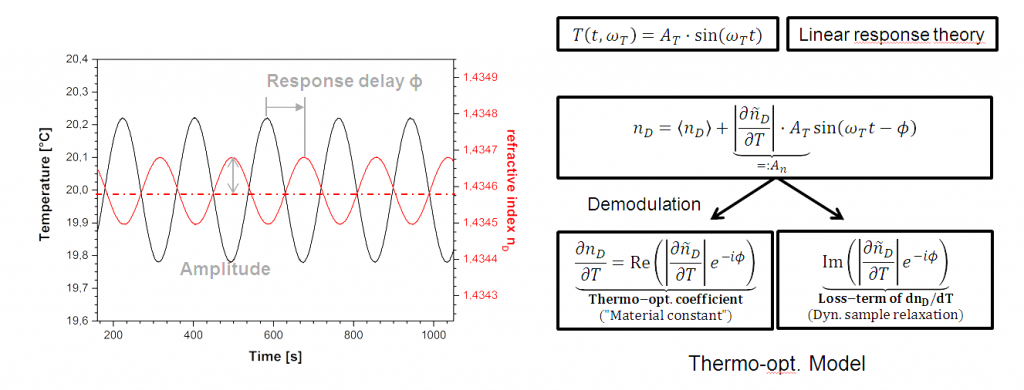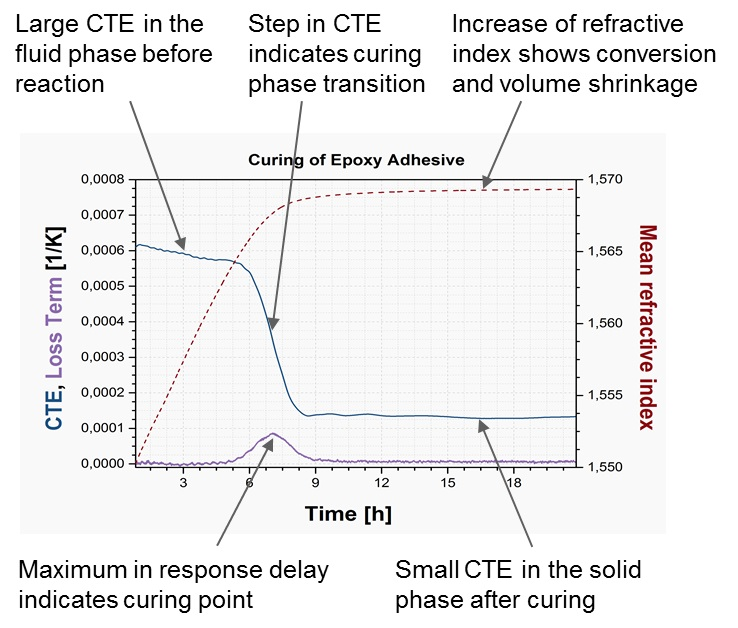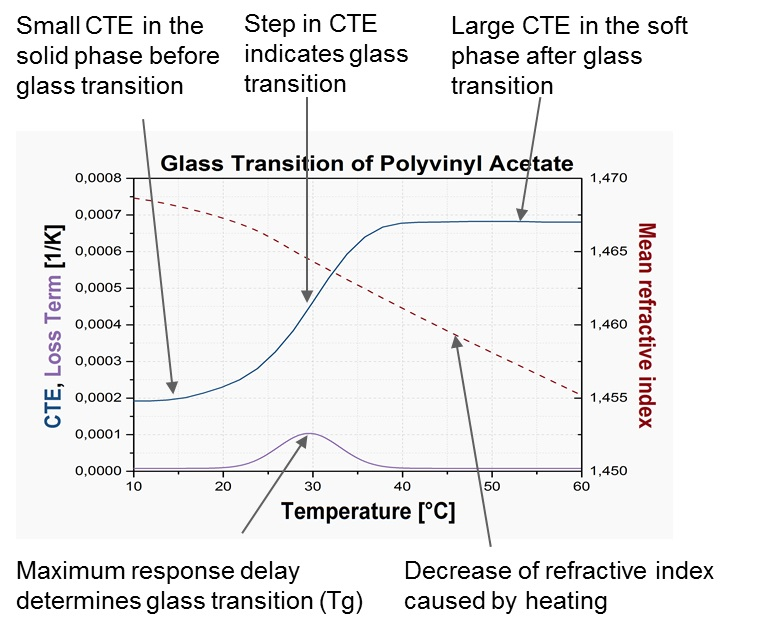3 Rates

# Thermal analysis based on an optical measurement: TORC

TORC is a novel thermal analysis technique based on an optical measurement. It is the short form for Thermo-optical Oscillating Refraction Characterization. The technique was developed by Anton Paar in cooperation with the group around Prof. Krüger at the University of Luxembourg. The TORC technique not only allows the determination of thermal transitions and the refractive index, it also enables the measurement of the coefficient of thermal expansion using mathematical models.

## Measurement principle

The TORC principle of thermal analysis is based on the measurement of a change in the optical response caused by thermal excitation.

### Thermal excitation – optical response

The TORC technique utilizes a minimal sinus-shaped temperature modulation. This small thermal excitation of around 0.1 K causes density and therefore volume changes. The measured optical response in the refractive index shows a modulation, too. This grants access to new parameters, the amplitude of the refractive index, and the phase shift/response delay between the temperature modulation and the refractive index modulation.Figure 1: Thermal analysis using the TORC technique

### Phase shift

The measurement of the phase shift  between the sinus wave of thermal excitation (temperature modulation) and the optical response (refractive index modulation) is used to determine changes inside the sample e.g. phase transitions. Without any change inside the sample the basic phase shift is around 180°. During changes like phase transitions or glass transitions the phase shift increases. The maximum of the phase shift can be utilized to determine e.g. the melting point. The phase shift is the basic parameter of the loss term calculation.

### Amplitude

The intensity of changes in the refractive index (amplitude, An) caused by the temperature modulation depends on the material. The measured material parameter is called dn/dT or the temperature-dependence of the refractive index. This value is the basis for the calculation of the coefficient of thermal expansion.

## Coefficient of thermal expansion

The thermal expansion coefficient is a measure of the increase or decrease of volume with temperature changes. The TORC technique utilizes mathematical models to make a connection between the refractive index and the density, which allows the calculation of the coefficient of thermal expansion. The basic connection between these two fundamental parameters is expressed by the Lorentz-Lorenz equation.

Substance Coefficient of thermal expansion [10⁻⁴/K] at 20 °C
Water 2.07
PS 2.1
PE (amorph) 8.7
Paraffin oil (low viscosity) 7.01
N-dodecane 7.19

Table 1: The volume coefficient of thermal expansion of different substances

### Lorentz-Lorenz equation

$$|{\widetilde{Y}}_{LL}|={{6 \cdot {\langle{n_D}\rangle}}\over(\langle{n_D}\rangle^{2}-1) \cdot (\langle{n_D}\rangle^{2}+2)}\cdot\left|{{d{\widetilde{n}}}_D}\over{dT}\right|$$

### Beysens model

The mathematical model based on Beysens is an improvement of the Lorentz-Lorenz equation used by investigating commonly used liquids.

$$|{\widetilde{Y}}_{Bey}|={{6 \cdot {\langle{n_D}\rangle}}\over(\langle{n_D}\rangle^{2}-1) \cdot (\langle{n_D}\rangle^{2}+2)}\cdot\left(1-{7\over8} \cdot{{{(\langle{n_D}\rangle^{2}-1)^{2}}}\over(\langle{n_D}\rangle^{2}+2)^{2}}\right)^{-1}\left|{{d{\widetilde{n}}}_D}\over{dT}\right|$$

## Result plot

The typical results plot shows the values for the coefficient of thermal expansion, the loss term and the mean refractive index plotted against the time or temperature.

### Result plot for time-dependent processes

This plot is used during time-dependent (also called quasi-isothermal) measurements. The result values are plotted against the time. This plot is commonly used to investigate curing, polymerization or ageing processes. Changes in the refractive index [red dotted line] can be used to determine in the reaction conversion or calculate the volume shrinkage. The maximum in the loss term indicates e.g. the curing point. Further, the coefficient of thermal expansion and its changes can be measured during the whole process. (Figure 2)Figure 2: Result plot for time-dependent processesFigure 3: Result plot for temperature-dependent processes

### Result plot for temperature-dependent processes

This plot is used for TORC measurement with temperature ramps. This plot is commonly used to investigate phase transitions like melting, crystallization, and glass transitions. The maximum in the loss term indicates e.g. the phase transition point. Further, the coefficient of thermal expansion and its changes can be measured during the whole process as well to determine e.g. the glass transition point. (Figure 3)

## Applications

The new TORC technique is commonly used in the investigation of time-dependent and temperature-dependent processes for thermal analysis, especially in the adhesive, polymer, and food processing industries.

### Adhesive and polymer industries

In these industries the TORC technique is used for:

• Quality control of raw, intermediate, and final products
• Determining the mixing ratio of multiple-component adhesives
• Determining the glass transition point of cured adhesives and polymers
• Investigating the influence of moisture, temperature, and mixture on curing process
• Investigating the influence of temperature and environmental conditions on ageing
• Monitoring polymerization
• Investigating volume shrinkage caused by curing or polymerization

### Food processing industry

In this industry the TORC technique is used for:

• Determining the melting point of fats and waxes
• Quality control of edible oils
• Determining a fat’s crystal modification via the melting point
• Determining the glass transition temperature as a function of water content in candies